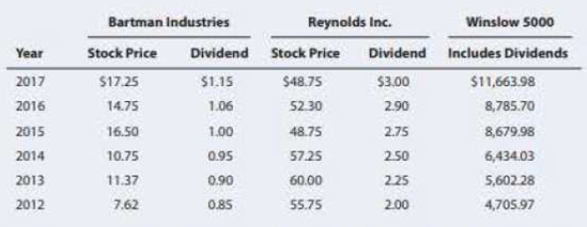Chapter 8, Problem 22SPFundamentals of Financial Manageme...

15th Edition
Eugene F. Brigham + 1 other
ISBN: 9781337395250

Solutions

Chapter
SectionFundamentals of Financial Manageme...

15th Edition
Eugene F. Brigham + 1 other
ISBN: 9781337395250
Textbook Problem

EVALUATING RISK AND RETURN Bartman Industrie's arul Reynolds Inc.'s stock prices and dividends, along with the Winslow 5000 Index, are shown here for the period 2012-2017. The Winslow 5000 data are adjusted to include dividends.a. Use the data to calculate annual rates of return for Bartman, Reynolds, and the Winslow 5000 Index Then calculate each entity's average return over the 5-year period. (Hint Remember, returns are calculated by subtracting the beginning price from the ending price to get the capital gain or loss, adding the dividend to the capital gain or toss, and dividing the result by the- beginning price. Assume that dividends are already included in the index. Also, you cannot calculate the rate of return for 2012 because you do not have 2011 data.) b. Calculate the standard deviations of the returns for Bartman, Reynolds, and the Winslow 5000. (Hint Use the sample standard deviation formula, Equation 8.2a in this chapter, which corresponds to the STDEV function in Excel. c. Calculate the coefficients of variation for Bartman, Reynolds, and the Winslow 5000. d. Assume the risk-free rate during this time was 3%. Calculate the Sharpe ratios for Bartram, Reynolds, and the Index over this period using their average returns. e. Construct a scatter diagram that shows Bartman's and Reynolds's returns on the vertical axis and the Winslow 5000 Index's returns on the horizontal axis. f. Estimate Bartman's and Reynolds's betas by running regressions of their returns against the index’s returns (Hint: Refer to Web Appendix 8A.) Are these betas consistent with your graph? g. Assume that the risk-free rate on long-term.Treasury bonds is 4.5 %. Assume also that the average annual return on the Winslow 5000 is not a good estimate of the market’s required return– it is too high. So use 10%. as the expected return on the market. Use the SML equation to calculate the two companies' required returns. h. If you formed a portfolio that consisted of 50% .Bartman and 50% Reynolds, what would the portfolio's beta and required return be? i. Suppose an investor wants to include Bartman Industries’s stock in his portfolio. Stocks A, B, and C are currently in the portfolio, and their betas ate 0.769, 0.985, and 1.423, respectively. Calculate the new portfolio's required return if it consists of 25% of Bartman, 15% ot Stock A,40% of Stock B, and 20% of Stock C.

a)

Summary Introduction

To determine: The annual rate of return and average return over 5 years’ period.

Introduction:

The risk and return are two closely related terms. The risk is the uncertainty attached to an event. In case of any investment, there is some amount of risk attached to it as there can be either gain or loss. While return in the financial term is that percentage which represents the profit in an investment.

Higher risk is associated with higher return and lower risk has a probability of lower return. The investor has to face a tradeoff between risk and return in terms of an investment.

The annual rate of return refers to that return which is charged or is earned on an investment for a year. This rate is expressed in percentage.

Explanation

Given information:

Refer table in the problem 8-22 in the text.

Calculate the annual rates of return:

b)

Summary Introduction

To prepare: The standard deviation for the given data.

Introduction:

The standard deviation refers to the stand-alone risk associated with the securities. It measures how much a data is dispersed with its standard value. The Greek letter sigma represents the standard deviation.

c)

Summary Introduction

To determine: The coefficient of variation.

Introduction:

The coefficient of variation is a tool to determine the investment’s volatility.

d)

Summary Introduction

To determine: Sharpe ratio

Introduction:

Sharpe ratio helps to determine the performance of the investment.

e)

Summary Introduction

To prepare: A scatter diagram showing the company’s returns and the index returns.

f)

Summary Introduction

To determine: The beta of the B Industries and R Inc. by running regressions of their returns.

g)

Summary Introduction

To determine: The required returns of the two companies by security market line equation.

h)

Summary Introduction

To determine: The beta and the required return for a newly constructed portfolio.

i)

Summary Introduction

To determine: The new portfolio’s required return.

Still sussing out bartleby?

Check out a sample textbook solution.

See a sample solution

The Solution to Your Study Problems

Bartleby provides explanations to thousands of textbook problems written by our experts, many with advanced degrees!

Get Started